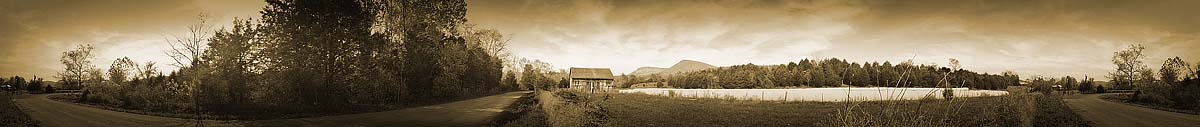H o g b a c k   M o u n t a i n   R o c k b r i d g e   C o u n t y
N e w  T o p o g r a p h i c s  O f  O l d  V i r g i n i a